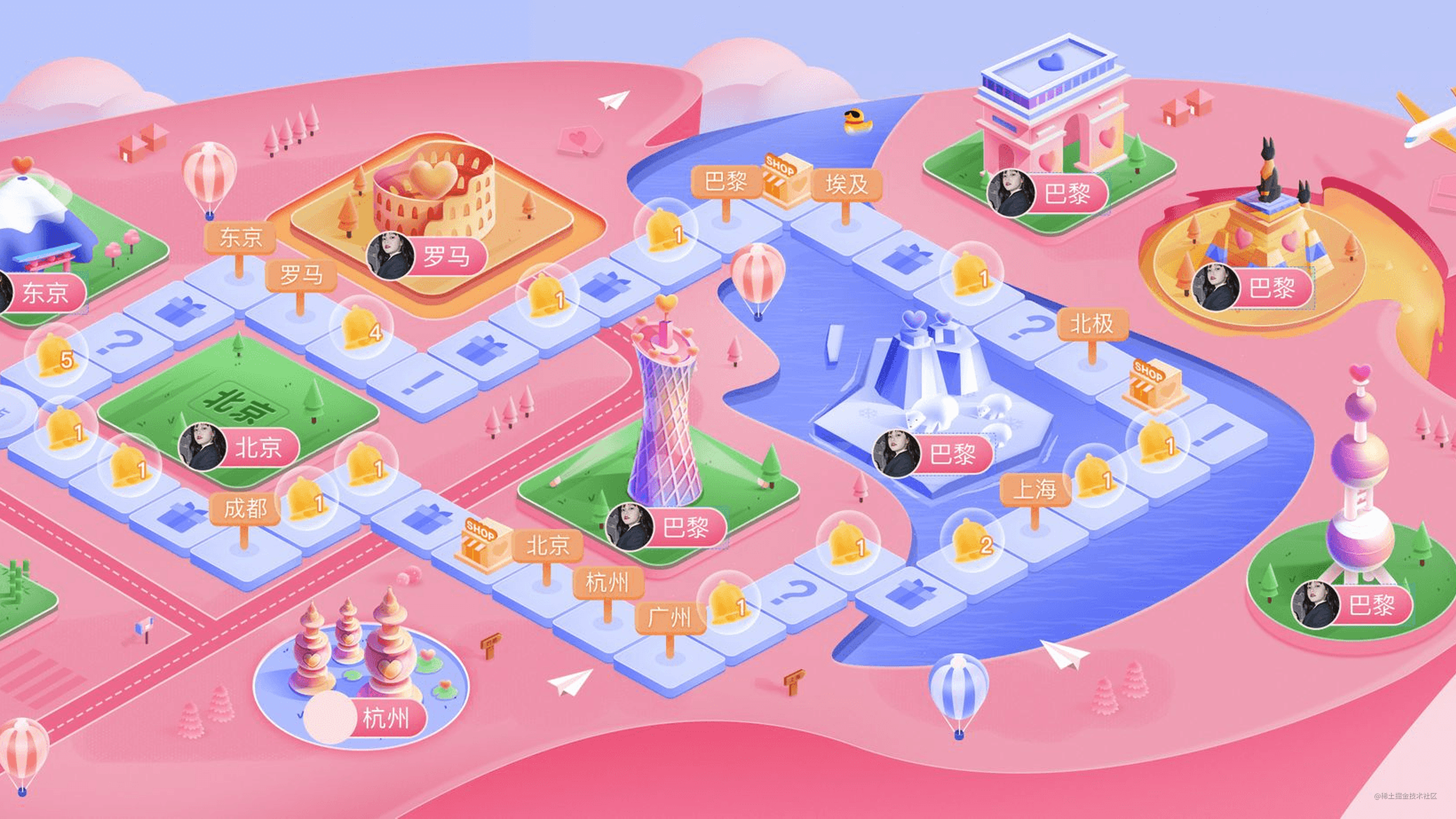# LOOK 直播活动地图生成器方案# 方案简述

## 位点图

1. 在每个方格左上角的位置，放置一个 1px 的像素点，不同类型的方格用不同颜色表示。
2. 底色为纯色：便于区分背景和方格。
3. 大小和地图背景图大小一致：便于从图中读出的坐标可以直接使用。## 读取位点图

``````const JImp = require('jimp');
const nodepath = require('path');

function parseImg(filename) {
JImp.read(filename, (err, image) => {
const { width, height } = image.bitmap;

const result = [];

// 图片左上角像素点的颜色, 也就是背景图的颜色
const mask = image.getPixelColor(0, 0);

// 筛选出非 mask 位置点
for (let y = 0; y < height; ++y) {
for (let x = 0; x < width; ++x) {
const color = image.getPixelColor(x, y);
if (mask !== color) {
result.push({
// x y 坐标
x,
y,
// 方格种类
type: color.toString(16).slice(0, -2),
});
}
}
}

// 输出
console.log(JSON.stringify({
// 路径
path: result,
}));
});
}

parseImg('bitmap.png');

``````{
"path": [
{
"type": "",
"x": 0,
"y": 0,
},
// ...
],
}

## 通路连通算法

``````function takePath(point, points) {
const candidate = (() => {
// 按照距离从小到大排序
const pp = [...points].filter((i) => i !== point);
const [one, two] = pp.sort((a, b) => measureLen(point, a) - measureLen(point, b));

if (!one) {
return [];
}

// 如果两个距离 比较小，则穷举两个路线，选择最短连通图路径。
if (two && measureLen(one, two) < 20000) {
return [one, two];
}
return [one];
})();

let min = Infinity;
let minPath = [];
for (let i = 0; i < candidate.length; ++i) {
// 递归找出最小路径
const subpath = takePath(candidate[i], removeItem(points, candidate[i]));

const path = [].concat(point, subpath);
// 测量路径总长度
const distance = measurePathDistance(path);

if (distance < min) {
min = distance;
minPath = subpath;
}
}

return [].concat(point, minPath);
}

# 方案优化

1. 只有 1px 的像素点太小了，肉眼无法辨别。不管是视觉同学还是开发同学，如果点错了位置就很难排查。
2. 位点图中包含的信息还是太少了，颜色仅仅对应种类，我们希望能够包含更多的信息，比如点之间的排列顺序、方格的大小等。

## 像素点合并1. 依次扫描图像中的像素点，当扫描到颜色与背景色不同的点时，记录下该点的坐标和颜色。2. 之后扫描与该点相邻的 8 个点，将这些点打上”已扫描“的标记。筛选出其中颜色与背景色不同且尚未被扫描过的点，放入待扫描的队列中。3. 从待扫描队列中取出下一个需要扫描的点，重复步骤 1 和步骤 2。

4. 直到待扫描的队列为空时，我们就扫描完了一整个有颜色的区域。区域合并完毕。``````const JImp = require('jimp');

let image = null;
let maskColor = null;

// 判断两个颜色是否为相同颜色 -> 为了处理图像颜色有误差的情况, 不采用相等来判断
const isDifferentColor = (color1, color2) => Math.abs(color1 - color2) > 0xf000ff;

// 判断是(x,y)是否超出边界
const isWithinImage = ({ x, y }) => x >= 0 && x < image.width && y >= 0 && y < image.height;

// 选择数量最多的颜色
const selectMostColor = (dotColors) => { /* ... */ };

// 选取左上角的坐标
const selectTopLeftDot = (reginDots) => { /* ... */ };

// 区域合并
const reginMerge = ({ x, y }) => {
const color = image.getPixelColor(x, y);
// 扫描过的点
const reginDots = [{ x, y, color }];
// 所有扫描过的点的颜色 -> 扫描完成后, 选择最多的色值作为这一区域的颜色
const dotColors = {};
dotColors[color] = 1;

for (let i = 0; i < reginDots.length; i++) {
const { x, y, color } = reginDots[i];

// 朝临近的八个个方向生长
const seeds = (() => {
const candinates = [/* 左、右、上、下、左上、左下、右上、右下 */];

return candinates
// 去除超出边界的点
.filter(isWithinImage)
// 获取每个点的颜色
.map(({ x, y }) => ({ x, y, color: image.getPixelColor(x, y) }))
// 去除和背景色颜色相近的点
.filter((item) => isDifferentColor(item.color, maskColor));
})();

for (const seed of seeds) {
const { x: seedX, y: seedY, color: seedColor } = seed;

// 将这些点添加到 reginDots, 作为下次扫描的边界
reginDots.push(seed);

// 将该点设置为背景色, 避免重复扫描

// 该点颜色为没有扫描到的新颜色, 将颜色增加到 dotColors 中
if (dotColors[seedColor]) {
dotColors[seedColor] += 1;
} else {
// 颜色为旧颜色, 增加颜色的 count 值
dotColors[seedColor] = 1;
}
}
}

// 扫描完成后, 选择数量最多的色值作为区域的颜色
const targetColor = selectMostColor(dotColors);

// 选择最左上角的坐标作为当前区域的坐标
const topLeftDot = selectTopLeftDot(reginDots);

return {
...topLeftDot,
color: targetColor,
};
};

const parseBitmap = (filename) => {
JImp.read(filename, (err, img) => {
const result = [];
const { width, height } = image.bitmap;
// 背景颜色
maskColor = image.getPixelColor(0, 0);
image = img;

for (let y = 0; y < height; ++y) {
for (let x = 0; x < width; ++x) {
const color = image.getPixelColor(x, y);

// 颜色不相近
if (isDifferentColor(color, maskColor)) {
// 开启种子生长程序, 依次扫描所有临近的色块
result.push(reginMerge({ x, y }));
}
}
}
});
};

## 颜色包含额外信息# 总结1. 视觉同学提供位点图，作为承载信息的载体，它需要满足以下三个要求：
1. 大小和地图背景图大小一致：便于我们从图中读出的坐标可以直接使用。
2. 底色为纯色：便于区分背景和方格。
3. 在每个方格左上角的位置，放置一个方格，不同颜色的方格表示不同类型。
2. 通过 `jimp` 扫描图片上每个像素点的颜色，从而生成一份包含各个方格位置和种类的 json。
3. 绘制地图时，先读取 json 文件，再根据 json 文件内的坐标信息和种类信息来放置素材。1. 由于 1px 的像素点对肉眼来说过小，视觉同学画图以及我们调试的时候，都十分不方便。因此我们将像素点扩大为一个区域，在扫描时，对相邻的相同颜色的像素点进行合并。
2. 让颜色的 rgba 分别对应一种信息，扩充位点图中的颜色值能够给我们提供的信息。

# FAQ

• 在位点图上，直接使用颜色块的大小作为路径方格的宽高可以不？

当然可以。但这种情况是有局限性的，当我们的素材很多且彼此重叠的时候，如果依然用方块大小作为宽高，那么在位点图上的方块就会彼此重叠，影响我们读取位置信息。

• 如何处理有损图的情况？

有损图中，图形边缘处的颜色和中心的颜色会略微有所差异。因此需要增加一个判断函数，只有扫描到的点的颜色与背景色的差值大于某个数字后，才认为是不同颜色的点，并开始区域合并。同时要注意在位点图中方块的颜色尽量选取与背景色色值相差较大的颜色。

这个判断函数，就是我们上面代码中的 isDifferentColor 函数。

``````const isDifferentColor = (color1, color2) => Math.abs(color1 - color2) > 0xf000ff;
复制代码``````
• 判断两个颜色不相等的 `0xf000ff` 是怎么来的？

随便定的。这个和图片里包含颜色有关系，如果你的背景色和图片上点的颜色非常相近的话，这个值就需要小一点；如果背景色和图上点的颜色相差比较大，这个值就可以大一点。